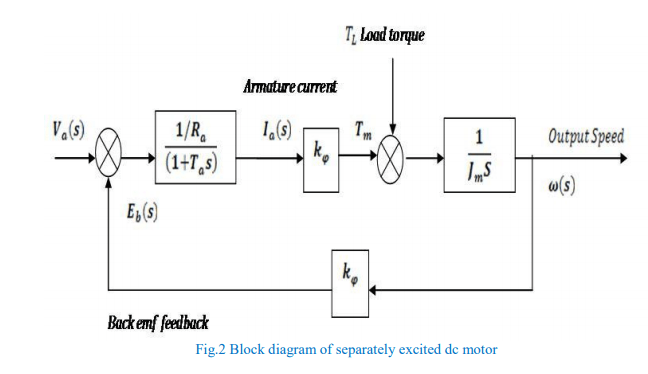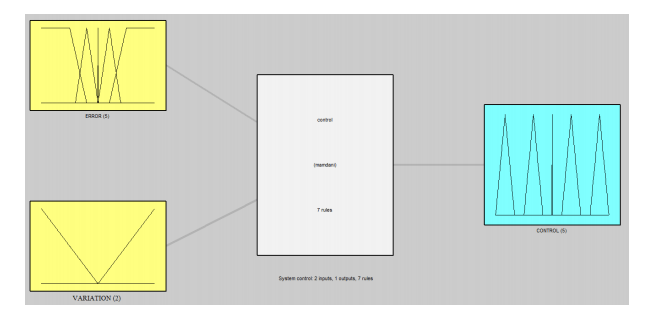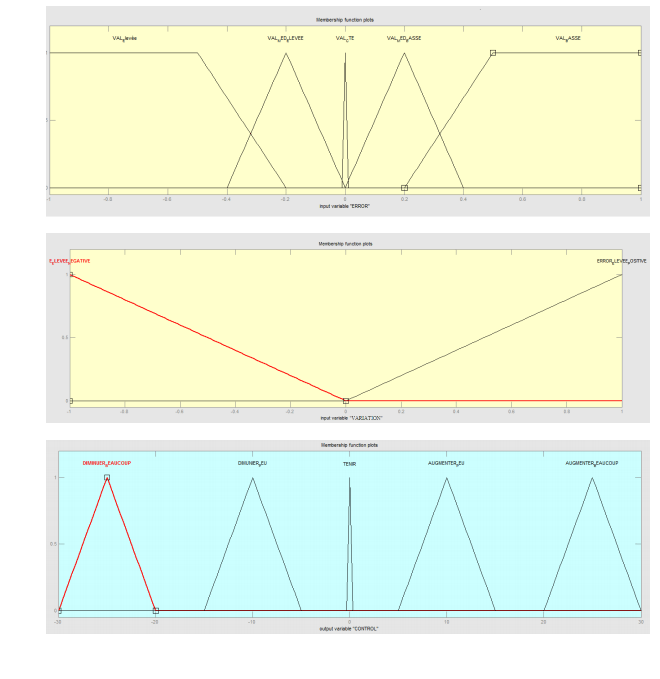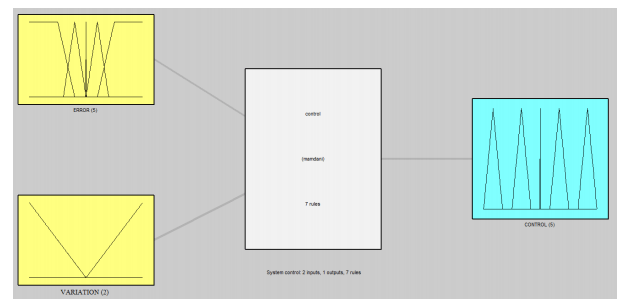# Fuzzy controller

EXERCISE: FUZZY CONTROLLER DESIGN

Part 1: design of the fuzzy controller

The objective of this case study is to perform the speed control of a separately excited DC motor (figure 1) using fuzzy logic controller (FLC). The controller will be designed based on the expert knowledge of the system. For the proposed dc motor case, we recommend 7 fuzzy rules for fuzzy logic controller.Taking field flux as Ø and back EMF constant as Kφ .

Equation fot back emf motor will be: Eb = Kφω ,  Torque: Tm = Jm(dω/dt)+TL     and     Tm = KφIa

ω is the angular velocity (speed) and friction in rotor of motor is very small (can be neglected) so Bm = 0.    Armature time constant : Ta = La/Ra

1) Plot the block diagram of separately excited dc motor based on Laplace transformations of the motor’s armature voltage and balance torque.

2) Define the required fuzzy controller inputs and outputs. Then complete this diagram:3) Deduce the structure of the fuzzy logic controller with closed loop (synopsis of all system with fuzzy controller).

4) Represent membership functions for inputs and output variables.

Input 1 range: [-1 1]

Input 2 range: [- 1 1]

Output range: [-30 30].

5) Enunciate the 7 “if-then” rules necessary for separately excited dc motor speed control.

6) What is the inference system type used here? Is there another type ?

7) What is the contribution (benefits) of fuzzy logic in comparison with a conventional PID controller for these case studies?

Part 2: Fuzzy controller implementation

We want to implement the proposed fuzzy controller under Matlab Simulink fuzzy logic toolbox.

1) What is the instruction to type on matlab to start the fuzzy logic toolbox?

2) Comment and give the output of every line of code in Matlab :

>> a = readfis(‘control.fis’)       >> getfis(a,’input’,1)     >> getfis(a,’output’,1)     >> plotfis(a)     >> mfedit(a)    >> ruleview(a)

CORRECTION:

Part 1:

1) Plot of the block diagram  based on Laplace transformations of the motor’s armature voltage and balance torque2) Definition of the required fuzzy controller inputs and outputs. diagram:3) Deduction of the structure of the fuzzy logic controller with closed loop (synopsis of all system with fuzzy controller).4) Representation of the membership functions for inputs and output variables.

Input 1 range: [-1 1]

Input 2 range: [- 1 1]

Output range: [-30 30].5) Enunciation of the 7  if_then rules necessary for separately excited  dc motor speed control.

-If (ERROR is VAL_BASSE) then (CONTROL is AUGMENTER_BEAUCOUP)

– If (ERROR is VAL_Elevée) then (CONTROL is DIMINUER_BEAUCOUP)

– If (ERROR is VAL_CTE) and (VARIATION is E_ELEVEE_NEGATIVE) then (CONTROL is DIMUNIER_PEU)

– If (ERROR is VAL_CTE) and (VARIATION is ERROR_ELEVEE_POSITIVE) then (CONTROL is AUGMENTER_PEU)

– If (ERROR is VAL_CTE) and (CAMBIO is ERROR_ELEVEE_POSITIVE) then (CONTROL is AUGMENTER_PEU)

– If (ERROR is VAL_MED_BASSE) then (CONTROL is AUGMENTER_PEU)

– If (ERROR is VAL_CTE) then (CONTROL is TENIR)

6) MANDANI. YES SUGENO METHOD

7) The fuzzy logic approach has minimum overshoot, minimum transient and steady state parameters, which shows more effectiveness and efficiency of FLC than conventional PID controller

Part 2:

1)The instruction to type on matlab to start the fuzzy logic toolbox is : Fuzzy

>> a = readfis(‘control.fis’) Creates a FIS matrix in the workspace corresponding to the FIS file ‘control’ on disk.

>> getfis(a,’input’,1) Gives the first input: ERROR

>> getfis(a,’output’,1) Gives the output: CONTROL

>> plotfis (a) Creates an input-output display of the fuzzy inference system associated    with the FIS structure FISSTRUCT>> mfedit(a) Generates a membership function editor that allows you to modify all the membership functions for your FIS stored in the file a.fis

>>ruleview(a) Opens the Rule Viewer, or Inference Diagram Viewer, for the fuzzy inference system, fis.